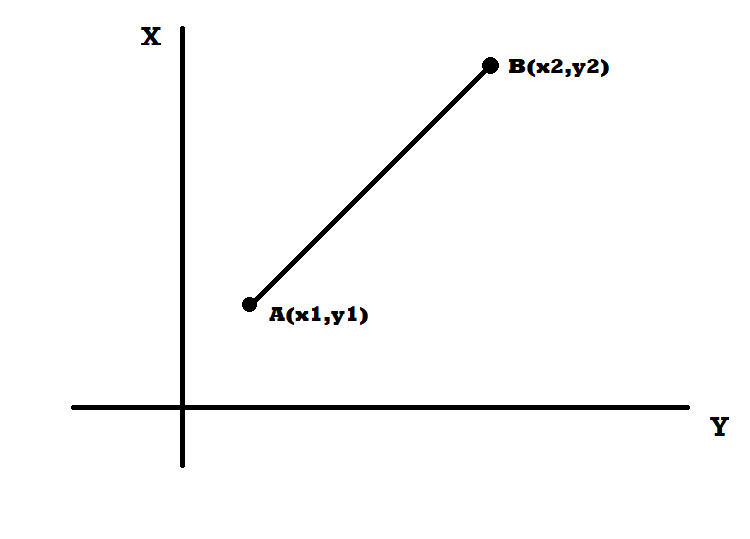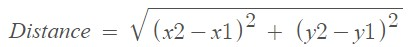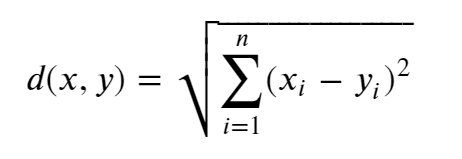Related Tags

python
machine learning
communitycreator

# How to calculate Euclidean distance

Irzum Jafri

### Background

Distance measures are an essential part of the machine learning algorithms.

Machine learning algorithms are divided into two types:

• Classification
• Regression

The regression algorithm learns from training data from labeled datasets, then predicts the output of test datasets based on the learning.

On the other hand, the classification algorithm differentiates between different objects in the dataset and creates categories (i.e., classifies) among them.

One example of a classification algorithm is the KNN algorithm.

KNN algorithms observe the distance between the training set data and test data, then utilize these distances to give the test point a label.

The nearer the test point is to a specific training point or a set of test points, the more likely it is to have a similar label as them.

To calculate these distances, we can use different methods. One of them is to calculate the Euclidean distance between these dataset points and use them in KNN algorithms.

The Euclidean distance measures clusters and non-metric multidimensional scaling, which was discovered by SIMPROF using the ANOSIMan analog of ANOVA randomization test (Clarke and Green, 1988).

### What is Euclidean distance?

Euclidean distance is simply the distance between two points. If the points are in a plane, then it is the distance of the segment connecting the two points. This distance is calculated by the Pythagoras theorem.

If we assume two points A(x1,y1) and B(x2,y2), then the Euclidean distance is shown in the graph below. This is also called straight line distance.The formula of Euclidean distance is given by:The formula for Euclidean distance in n points is given by:The Euclidean distance in machine learning can be calculated from the following code:

def euclidean_distance(A,B):
dist = np.linalg.norm(A - B)
return dist

### Properties

The Euclidean distance is an example of distance in metric space, so it obeys all of its properties:

• It is symmetric, which means that for all points A and B, Distance(A,B) = Distance(B,A)
• The Euclidean distance is always positive.
• It also obeys the triangle inequality law, which is stated below: for points a, b and c, dist(a,b) + d(b,c) >= dist(a,c)

RELATED TAGS

python
machine learning
communitycreator

CONTRIBUTOR

Irzum Jafri
RELATED COURSES

View all Courses

Keep Exploring

Learn in-demand tech skills in half the time